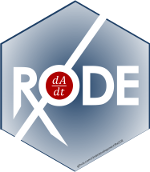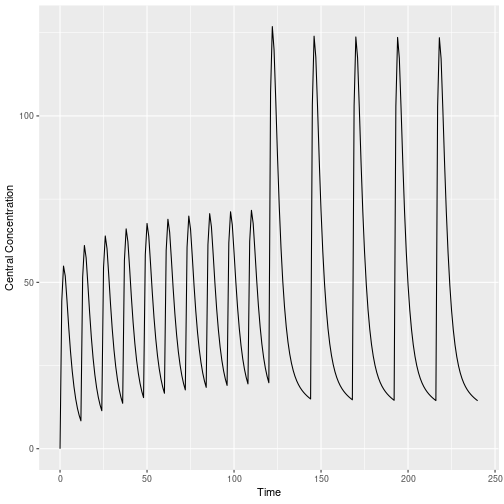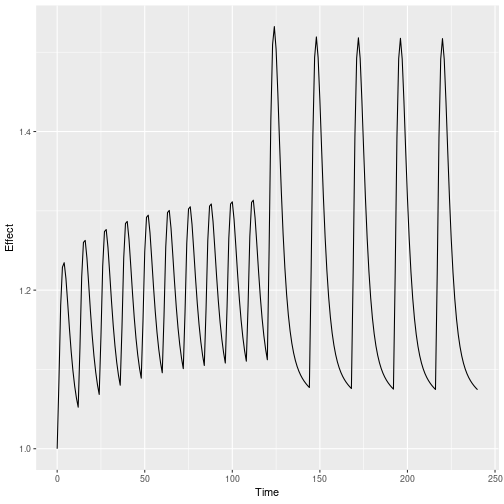<!–html_preserve–><!–/html_preserve–>

# Introduction

`RxODE` is an R package that facilitates simulation with ODE models in R. It is designed with pharmacometrics models in mind, but can be applied more generally to any ODE model.

# Description of RxODE illustrated through an example

The model equations can be specified through a text string, a model file or an R expression. Both differential and algebraic equations are permitted. Differential equations are specified by `d/dt(var_name) =`. Each equation can be separated by a semicolon.

To load `RxODE` package and compile the model:

``````library(RxODE)
mod1 <-RxODE({
C2 = centr/V2;
C3 = peri/V3;
d/dt(depot) =-KA*depot;
d/dt(centr) = KA*depot - CL*C2 - Q*C2 + Q*C3;
d/dt(peri)  =                    Q*C2 - Q*C3;
d/dt(eff)  = Kin - Kout*(1-C2/(EC50+C2))*eff;
});
``````

## Specify ODE parameters and initial conditions

Model parameters can be defined as named vectors. Names of parameters in the vector must be a superset of parameters in the ODE model, and the order of parameters within the vector is not important.

``````theta <-
c(KA=2.94E-01, CL=1.86E+01, V2=4.02E+01, # central
Q=1.05E+01,  V3=2.97E+02,              # peripheral
Kin=1, Kout=1, EC50=200)               # effects
``````

Initial conditions (ICs) are defined through a vector as well. If the elements are not specified, the initial condition for the compartment is assumed to be zero.

``````inits <- c(eff=1);
``````

## Specify Dosing and sampling in RxODE

`RxODE` provides a simple and very flexible way to specify dosing and sampling through functions that generate an event table. First, an empty event table is generated through the “eventTable()” function:

``````ev <- eventTable(amount.units='mg', time.units='hours')
``````

Next, use the `add.dosing()` and `add.sampling()` functions of the `EventTable` object to specify the dosing (amounts, frequency and/or times, etc.) and observation times at which to sample the state of the system. These functions can be called multiple times to specify more complex dosing or sampling regiments. Here, these functions are used to specify 10mg BID dosing for 5 days, followed by 20mg QD dosing for 5 days:

``````ev\$add.dosing(dose=10000, nbr.doses=10, dosing.interval=12)
``````

If you wish you can also do this with the `mattigr` pipe operator `%>%`

``````ev <- eventTable(amount.units="mg", time.units="hours") %>%
``````

The functions `get.dosing()` and `get.sampling()` can be used to retrieve information from the event table.

``````knitr::kable(head(ev\$get.dosing()))
``````
time evid amt
0 101 10000
12 101 10000
24 101 10000
36 101 10000
48 101 10000
60 101 10000
``````knitr::kable(head(ev\$get.sampling()))
``````
time evid amt
16 0 0 NA
17 1 0 NA
18 2 0 NA
19 3 0 NA
20 4 0 NA
21 5 0 NA

# Solving ODEs

The ODE can now be solved by calling the model object's `run` or `solve` function. Simulation results for all variables in the model are stored in the output matrix x.

You can also solve this and create a RxODE data frame:

``````x <- solve(mod1,theta, ev, inits);
rxHtml(x)
``````

Solved RxODE object
Parameters (\$params):
V2 V3 KA CL Q Kin Kout EC50
40.2 297 0.294 18.6 10.5 1 1 200
Initial Conditions ( \$inits):
depot centr peri eff
0 0 0 1
First part of data (object):
time C2 C3 depot centr peri eff
0 0.00000 0.0000000 10000.000 0.000 0.0000 1.000000
1 44.37555 0.9198298 7452.765 1783.897 273.1895 1.084664
2 54.88296 2.6729825 5554.370 2206.295 793.8758 1.180825
3 51.90343 4.4564927 4139.542 2086.518 1323.5783 1.228914
4 44.49738 5.9807076 3085.103 1788.795 1776.2702 1.234610
5 36.48434 7.1774981 2299.255 1466.670 2131.7169 1.214742

``````x <- mod1\$solve(theta, ev, inits)
``````
time C2 C3 depot centr peri eff
0 0.00000 0.0000000 10000.000 0.000 0.0000 1.000000
1 44.37555 0.9198298 7452.765 1783.897 273.1895 1.084664
2 54.88296 2.6729825 5554.370 2206.295 793.8758 1.180825
3 51.90343 4.4564927 4139.542 2086.518 1323.5783 1.228914
4 44.49738 5.9807076 3085.103 1788.795 1776.2702 1.234610
5 36.48434 7.1774981 2299.255 1466.670 2131.7169 1.214742

This returns a matrix. You can see the compartment values in the plot below:

``````library(ggplot2)
x <- as.data.frame(x)
ggplot(x,aes(time,C2)) + geom_line() + ylab("Central Concentration") + xlab("Time");
````````````ggplot(x,aes(time,eff)) + geom_line() + ylab("Effect") + xlab("Time");
``````